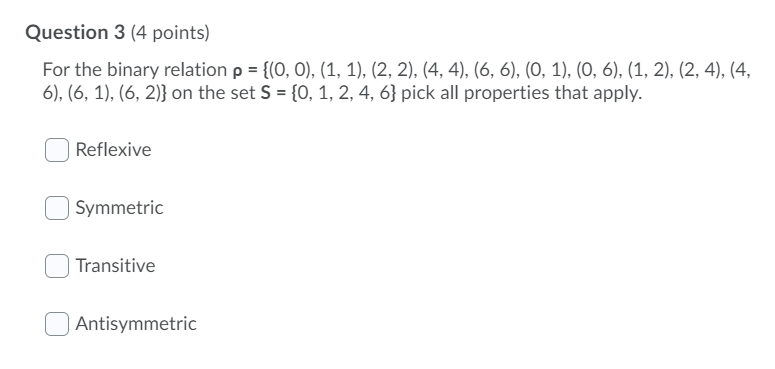# Question Question 3 (4 points) For the binary relation p = {(0, 0), (1, 1), (2, 2), (4,4), (6, 6), (0, 1), (0, 6), (1, 2), (2, 4), (4, 6), (6, 1), (6, 2)} on the set S = {0, 1, 2, 4, 6} pick all properties that apply. Reflexive Symmetric Transitive AntisymmetricGWV7SH The Asker · Computer ScienceTranscribed Image Text: Question 3 (4 points) For the binary relation p = {(0, 0), (1, 1), (2, 2), (4,4), (6, 6), (0, 1), (0, 6), (1, 2), (2, 4), (4, 6), (6, 1), (6, 2)} on the set S = {0, 1, 2, 4, 6} pick all properties that apply. Reflexive Symmetric Transitive Antisymmetric
More
Transcribed Image Text: Question 3 (4 points) For the binary relation p = {(0, 0), (1, 1), (2, 2), (4,4), (6, 6), (0, 1), (0, 6), (1, 2), (2, 4), (4, 6), (6, 1), (6, 2)} on the set S = {0, 1, 2, 4, 6} pick all properties that apply. Reflexive Symmetric Transitive Antisymmetric